Mean and Range

Chapter 3 Class 7 Data Handling
Concept wise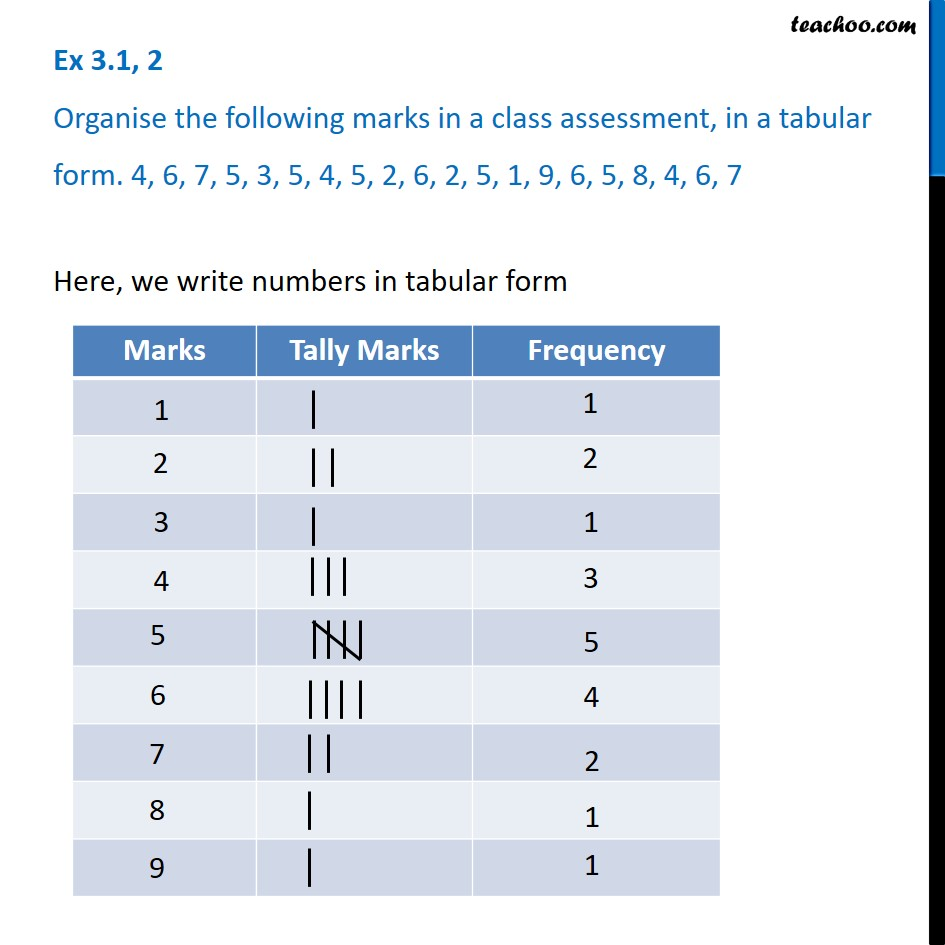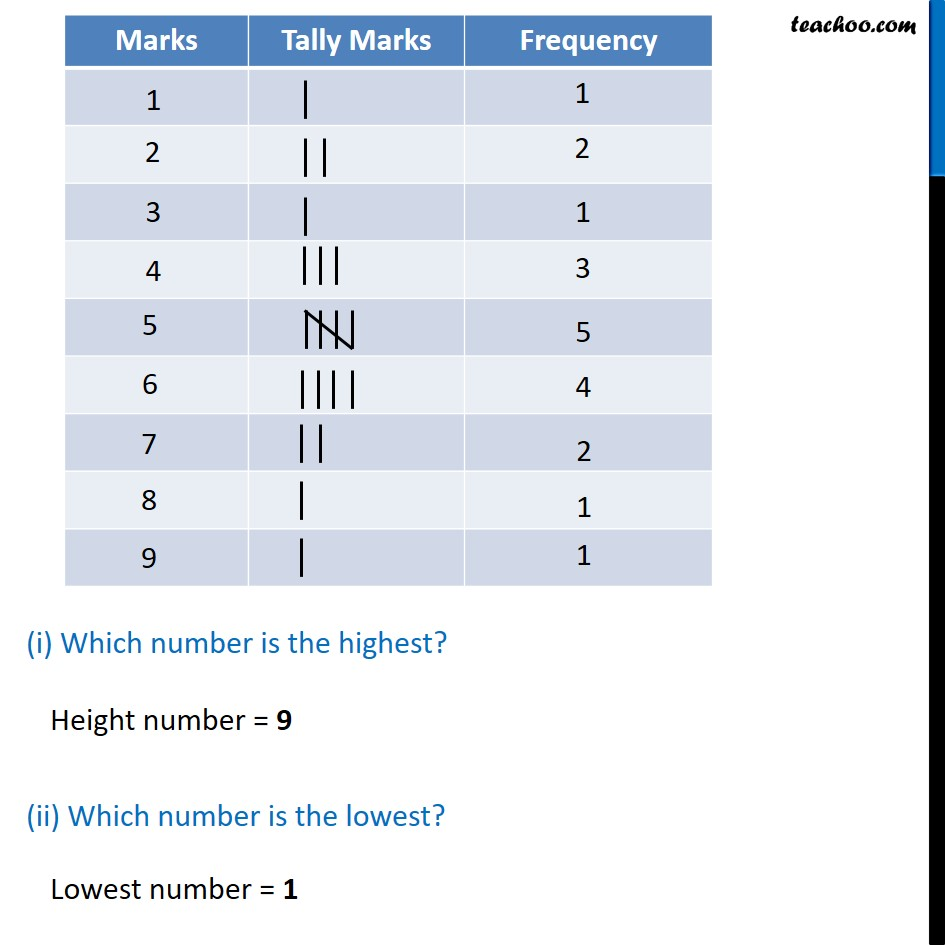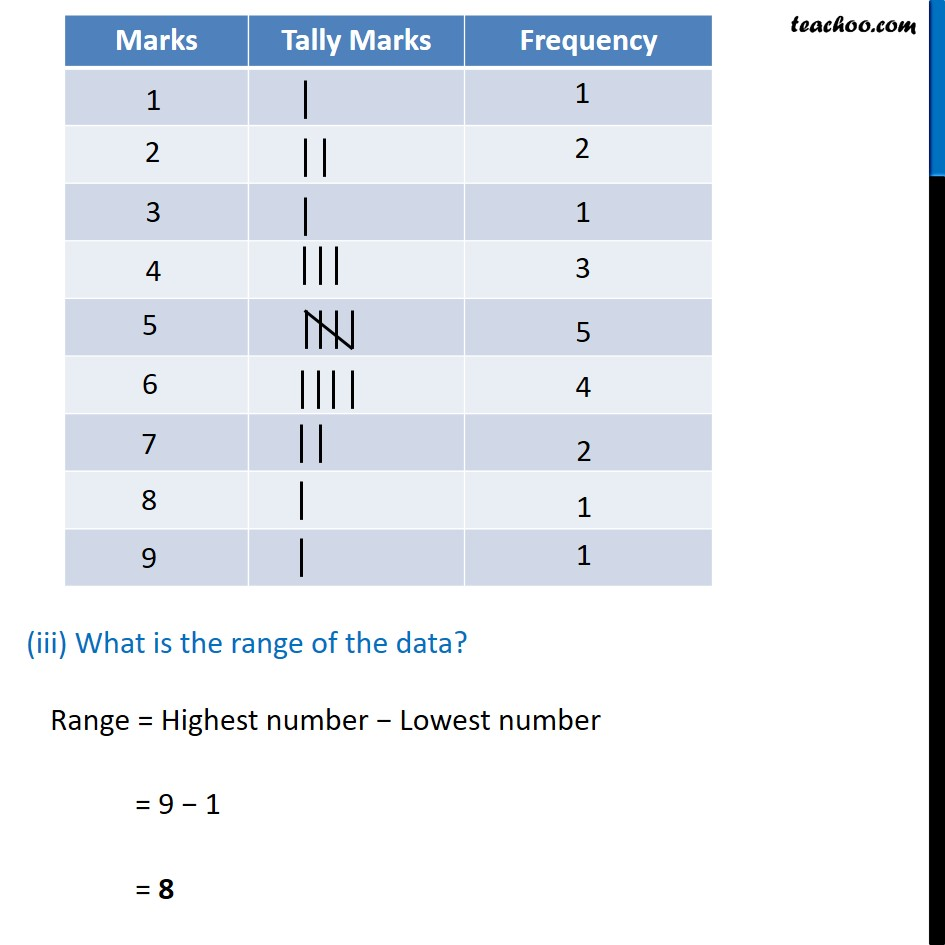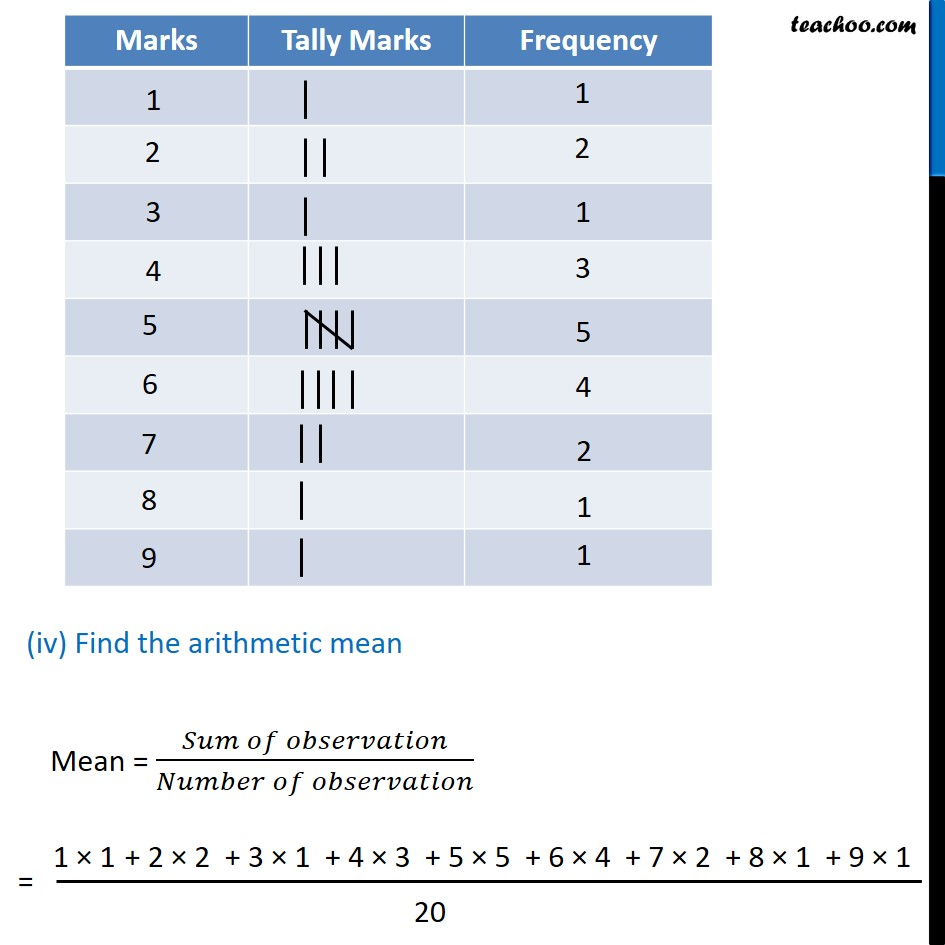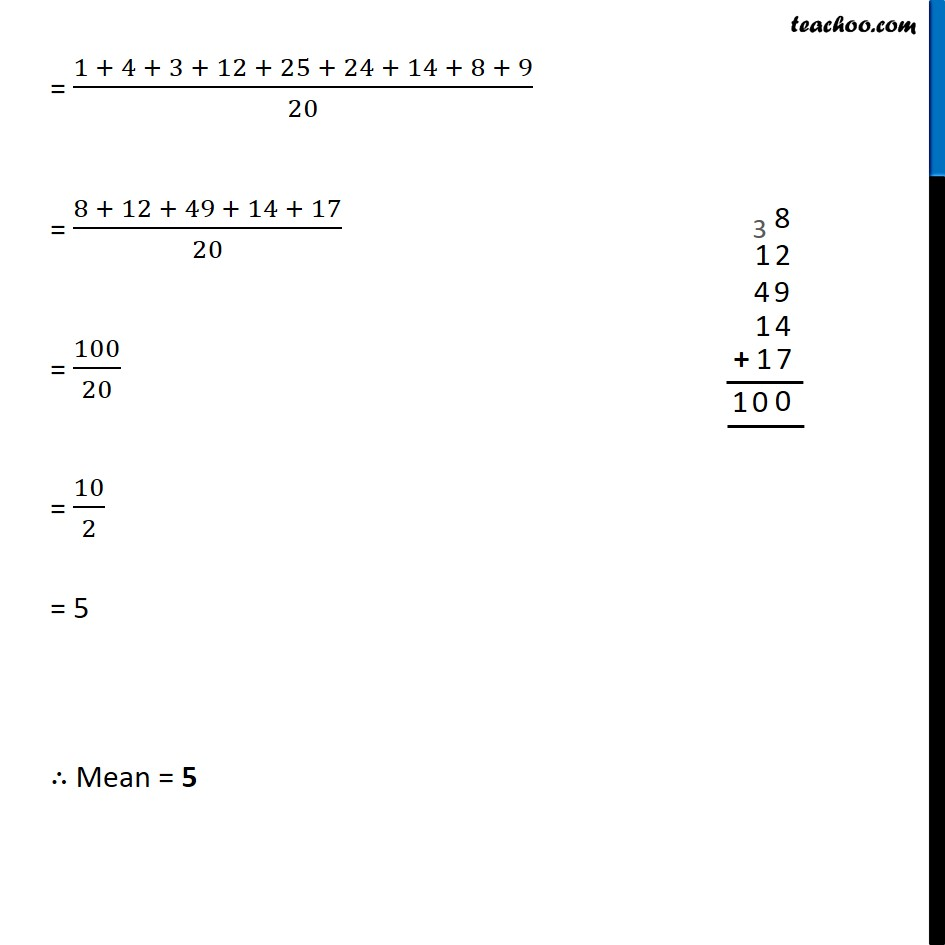Learn in your speed, with individual attention - Teachoo Maths 1-on-1 Class

### Transcript

Ex 3.1, 2 Organise the following marks in a class assessment, in a tabular form. 4, 6, 7, 5, 3, 5, 4, 5, 2, 6, 2, 5, 1, 9, 6, 5, 8, 4, 6, 7 Here, we write numbers in tabular form (i) Which number is the highest? (ii) Which number is the lowest? Height number = 9 Lowest number = 1 (iii) What is the range of the data? Range = Highest number − Lowest number = 9 − 1 = 8 (iv) Find the arithmetic mean Mean = (𝑆𝑢𝑚 𝑜𝑓 𝑜𝑏𝑠𝑒𝑟𝑣𝑎𝑡𝑖𝑜𝑛)/(𝑁𝑢𝑚𝑏𝑒𝑟 𝑜𝑓 𝑜𝑏𝑠𝑒𝑟𝑣𝑎𝑡𝑖𝑜𝑛) = (𝟏 + 𝟒 + 𝟑 + 𝟏𝟐 + 𝟐𝟓 + 𝟐𝟒 + 𝟏𝟒 + 𝟖 + 𝟗)/𝟐𝟎 = (𝟖 + 𝟏𝟐 + 𝟒𝟗 + 𝟏𝟒 + 𝟏𝟕)/𝟐𝟎 = 𝟏𝟎𝟎/𝟐𝟎 = 𝟏𝟎/𝟐 = 5 ∴ Mean = 5